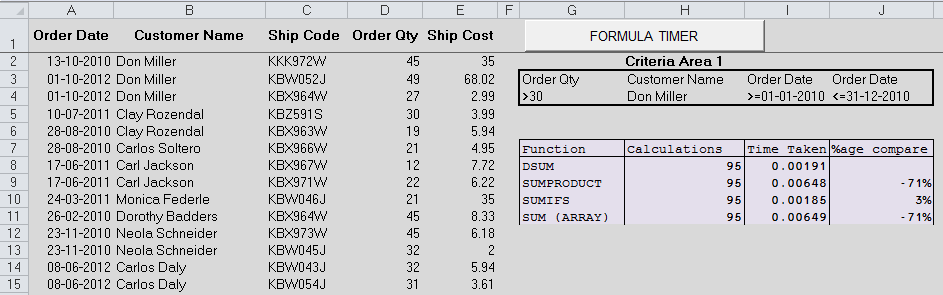# EXCEL DSUM FUNCTION

If you are new to Database Functions, read this Introduction to Database Functions first before you continue.

In this article we shall look into the uses of DSUM function which is one of the EFFICIENT Excel sum functions with criteria.

Below are some of its uses;

1. 2 way lookup
2. Summarizing Results based on criteria

# 2 WAY LOOKUP USING DSUM

Suppose you have below sales forecast  and you want to lookup the forecast of Papers in the Month of April`=DSUM(A2:G19,"Apr",I2:I3) = 218`
• A2:G19 = Database
• “Apr” = Field
• I2:I3 =Criteria

►How about sales forecast of  Papers & Tables for the month of April?

Just adjust the criteria area to create Paper OR Table Lookup►Now how about sales forecast of Papers & Tables  for the month of March &  April ?

DSUM is limited in this case as it only accepts ONE specified Field.

So, you need to Combine two  DSUM functions

`=DSUM(A2:G19,"Apr",I2:I4)+DSUM(A2:G19,"Mar",I2:I4) =292.02`

A better option for this kind of 2 way lookup is to use SUMPRODUCT

`=SUMPRODUCT(((B2:G2="Mar")+(B2:G2="Apr"))*((A3:A19="Paper")+(A3:A19="Tables"))*B3:G19) =292.02`

Or Use an Array SUM Function below

`{=SUM(D3:E19*((A3:A19="Paper")+(A3:A19="Tables")))}`

NB: For the above array formula, Just select the Months of  march & April (D3:E19) and NOT the whole database.

## How Does DSUM Compare To Other 2 Way Lookup Function

I have done the calculations and below are the results. DSUM is the fastest 2 way lookup  function# Summarizing Results Based On Criteria

In this example, we are going to use a worksheet with over 8000 rows so that we can also compare the speed of DSUM with other Sum functions like AGGREGATE, SUMPRODUCT & SUMIFS

so, to follow on with the example, Please DOWNLOAD WORKSHEET

Supposing you want to sum the orders for Don Miller which are for the Year 2010 which were greater than 30 units?Solution:

Set up a criteria area as shown in this article  to include all the values to be met and write below formula

`=DSUM(Orders,"Order Qty",G3:J4)`

NB:

• Orders►Named range = A1:E8400
• Incase you want to create more summaries, just adjust the criteria area & make it as COMPLEX as possible.

## Comparison of DSUM to other Excel Sum function

So, Is DSUM the fastest among the excel sum functions?

With the guidance from this Video I have created below summary which shows that  although DSUM is 71% Faster than SUMPRODUCT or array function SUM, it is 3% slower than SUMIFS.

Although SUMIFS is faster, it fails in handling complex criteria. So, DSUM (in my opinion) reigns  among the Sum functions.DOWNLOAD WORKSHEET

### RELATED ARTICLES

INTRODUCTION TO DATABASE FUNCTIONS

2 WAY LOOKUP IN EXCEL